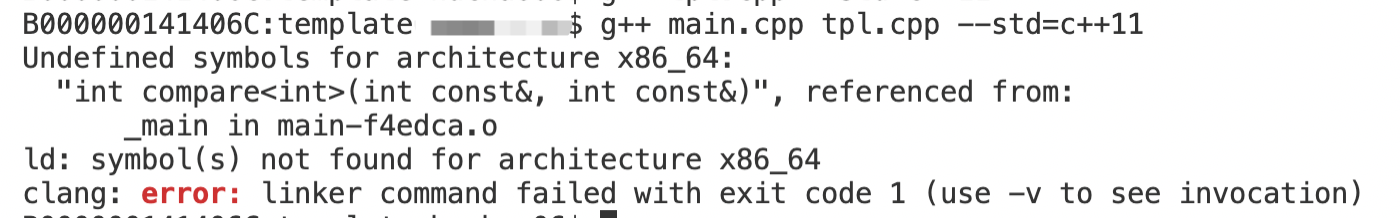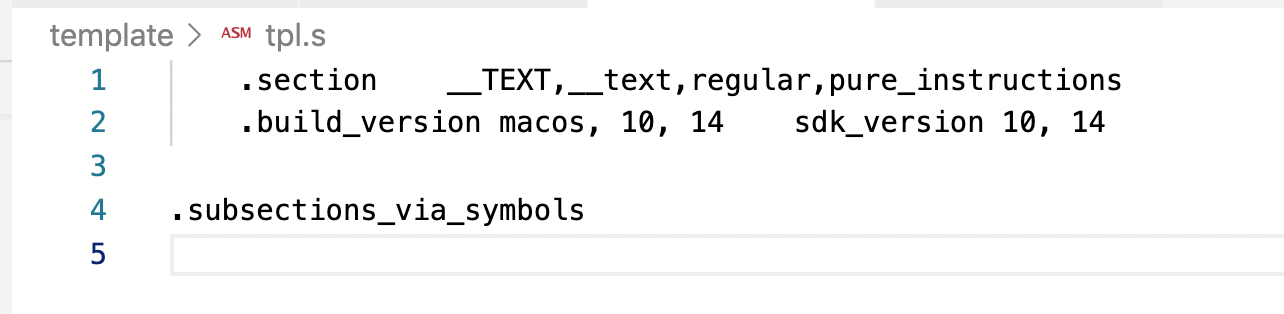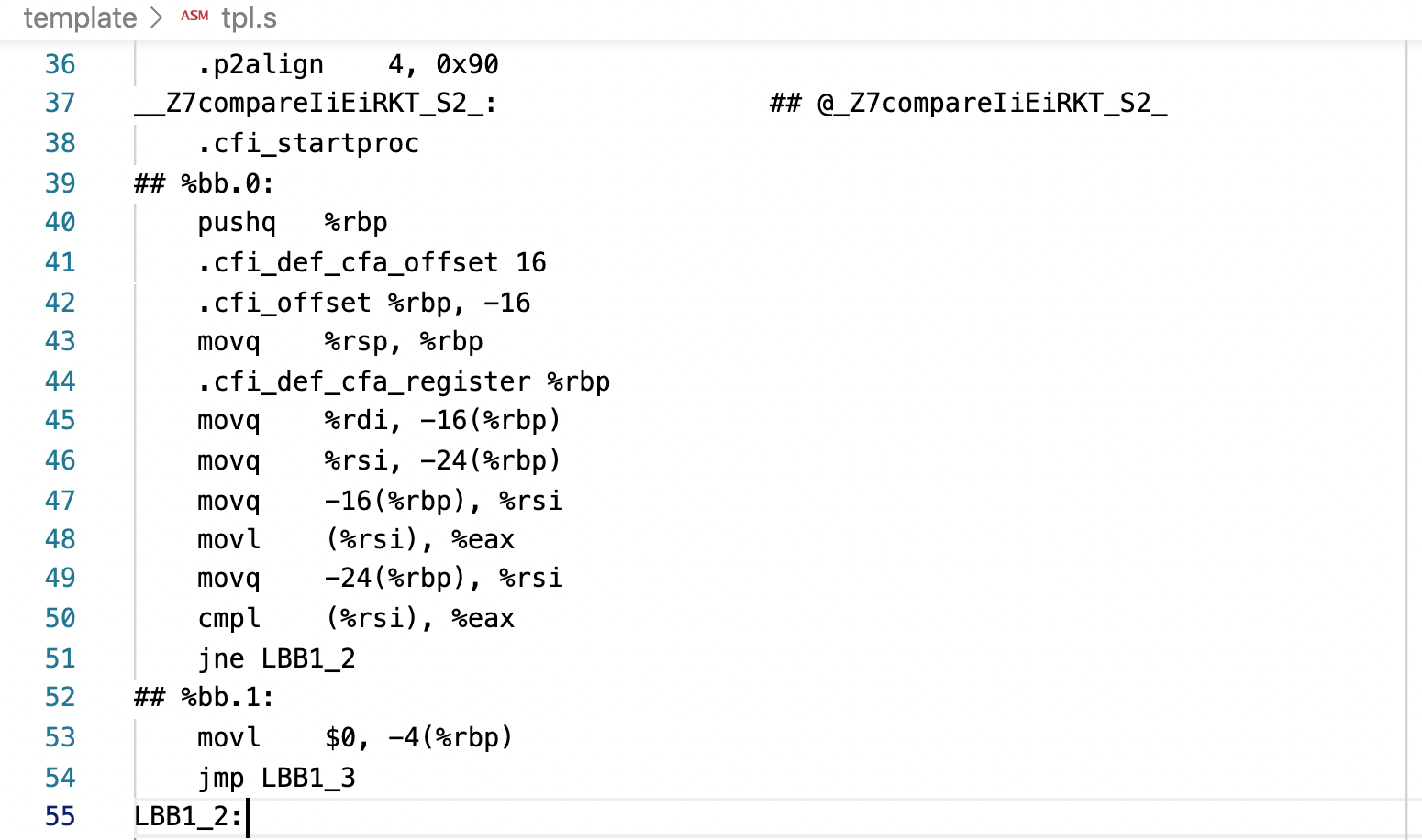# 为什么C++模板不支持分离式编译

## 前言图一

``````#pragma once

template <typename T>
int compare(const T& a, const T& b);``````

tpl.cpp代码如下：

``````#include "tpl.h"
#include <iostream>

template <typename T>
int compare(const T& a, const T& b) {
if (a == b) {
return 0;
}
return (a > b) ? 1 : -1;
}``````

main.cpp代码如下：

``````#include <iostream>
#include "tpl.h"

int main() {
int res = compare<int> (1, 2);
std::cout << res << std::endl;
}``````

## 模板的编译图二

``````int test() {
int res = compare<int> (1, 2);
std::cout << res << std::endl;
return res;
}``````图三

## 链接错误问题分析

1. 编译并且汇编tpl.cpp，生成tpl.o目标文件。
2. 编译并且汇编main.cpp，生成main.o目标文件。
3. 链接tpl.o和main.o生成可执行文件。

## 如何改正

``````#pragma once

template <typename T>
int compare(const T& a, const T& b) {
if (a == b) {
return 0;
}
return (a > b) ? 1 : -1;
}
``````微信赞赏支付宝赞赏